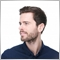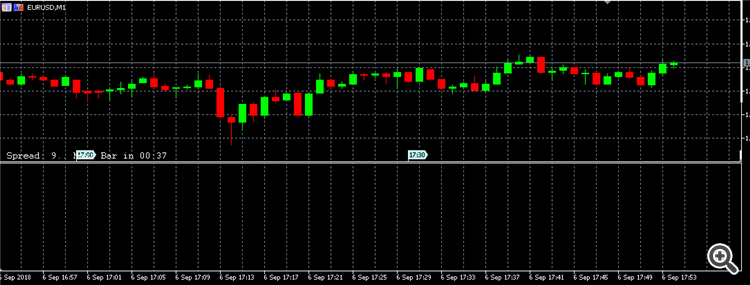# Candles not getting displayed from the file.3596

I have tried the following method to display the candles from a a file to the indicator window. See the code:

```#property version "1.10"
// ---- drawing the indicator in a separate window
#property indicator_separate_window
// ----, eight buffers are used to calculate and draw the indicator
#property indicator_buffers 8
// ---- used only four graphical constructions
#property indicator_plots 4

#property indicator_type1 DRAW_COLOR_CANDLES
//#property indicator_color4 clrDodgerBlue, clrLightSkyBlue, clrGray, clrPink, clrMagenta
#property indicator_color1 clrLime, clrRed, clrGray
// ---- display indicator label
#property indicator_label1 "Open; High; Low; Close"

input int Shift = 0; // shift the indicator lights horizontally in bars to the left

double ExtOpenBuffer [];
double ExtHighBuffer [];
double ExtLowBuffer [];
double ExtCloseBuffer [];
double ExtColorsBuffer [];

int min_rates_total, min_rates_1, min_rates_2, min_rates_3;
// ---- declaration of global variables
double o[],h[],l[],c[];
// + ----------------------------------------------- ------------------- +
// | Recalculate the position of the newest element in the array |
// + ----------------------------------------------- ------------------- +

string filename = "traincandle.csv",all,lines[],cell[];
ushort SepLine = StringGetCharacter("\n",0),
SepCell = StringGetCharacter(",",0);
int OnInit ()
{
int nL,i,hdl = FileOpen(filename,FILE_READ|FILE_WRITE|FILE_BIN|FILE_COMMON); // |FILE_COMMON: a csv-file in the common folder
if (hdl<0)
{
Alert("Can't open ",filename,", err: ",_LastError);
}
int sz = (int)FileSize(hdl);
all = FileReadString(hdl,sz); // read complete file

nL = i = StringSplit(all,SepLine,lines); // split into lines => \n is eliminated!!
ArrayResize(o,i);
ArrayResize(h,i);
ArrayResize(l,i);
ArrayResize(c,i);
int start = -1;
while (start++ < i-2) { // read from last to first line
int nC = StringSplit(lines[start],SepCell,cell);
o[start] = StringToDouble(cell);
h[start] = StringToDouble(cell);
l[start] = StringToDouble(cell);
c[start] = StringToDouble(cell);
}
ArrayPrint(cell);
ArraySetAsSeries(o,true);
ArraySetAsSeries(h,true);
ArraySetAsSeries(l,true);
ArraySetAsSeries(c,true);

FileClose(hdl);

SetIndexBuffer (0, ExtOpenBuffer, INDICATOR_DATA);
SetIndexBuffer (1, ExtHighBuffer, INDICATOR_DATA);
SetIndexBuffer (2, ExtLowBuffer, INDICATOR_DATA);
SetIndexBuffer (3, ExtCloseBuffer, INDICATOR_DATA);
SetIndexBuffer (4, ExtColorsBuffer, INDICATOR_COLOR_INDEX);
PlotIndexSetInteger (0, PLOT_SHOW_DATA, false);
PlotIndexSetInteger (1, PLOT_SHOW_DATA, false);
PlotIndexSetInteger (2, PLOT_SHOW_DATA, false);

PlotIndexSetInteger (3, PLOT_SHOW_DATA, false);
ArraySetAsSeries (ExtOpenBuffer, true);
ArraySetAsSeries (ExtHighBuffer, true);
ArraySetAsSeries (ExtLowBuffer, true);
ArraySetAsSeries (ExtCloseBuffer, true);
ArraySetAsSeries (ExtColorsBuffer, true);

IndicatorSetInteger (INDICATOR_DIGITS, _Digits);
string short_name = "Training";
IndicatorSetString (INDICATOR_SHORTNAME, short_name);
return (INIT_SUCCEEDED);
}
int OnCalculate (const int rates_total,
const int prev_calculated,
const datetime & time [],
const double & open [],
const double & high [],
const double & low [],
const double & close [],
const long & tick_volume [],
const long & volume [],
const int & spread [])
{
if (rates_total <min_rates_total) return (0);

int bar, limit;

if (prev_calculated> rates_total || prev_calculated <= 0) // check for the first start of the indicator calculation
{
limit = rates_total-2;
}
else
{
limit = rates_total-prev_calculated; // starting number for calculating new bars
}
//ArrayFill(ExtOpenBuffer,0,limit,0);
//ArrayFill(ExtHighBuffer,0,limit,0);
//ArrayFill(ExtLowBuffer,0,limit,0);
//ArrayFill(ExtCloseBuffer,0,limit,0);

// ---- the main cycle of calculation of the candlestick indicator
for (bar = ArraySize(o)-1; bar>=0 &&! IsStopped (); bar--)
{
// ----
ExtOpenBuffer [bar] = o[bar];
ExtHighBuffer [bar] = h[bar];
ExtLowBuffer [bar] = l[bar];
ExtCloseBuffer [bar] = c[bar];
if(o[bar] < c[bar])
ExtColorsBuffer[bar] = 0;
else if(o[bar] > c[bar])
ExtColorsBuffer[bar] = 1;
else
ExtColorsBuffer[bar] = 2;

}

return (1);
}
```

I have attached the sample file that I have used in the indicator.

I am not able to see the candles on the screen see the image:Kindly, help me get the values displayed on the indicator window. Please let me know how to show it as it is while time move on.

Files: